# Domains and Ranges of Standard Functions Set 3

Go back to  'Functions'

11. Trigonometric functions

There are six basic trigonometric functions as you know. Here, we will discuss how we can arrive at their graphs.

To draw them, we use the device of a unit circle and a point P moving in an anticlockwise direction on the circumference of this circle as shown in Fig - 18. ‘x’ is the angle in radians that OP makes with the x-axis.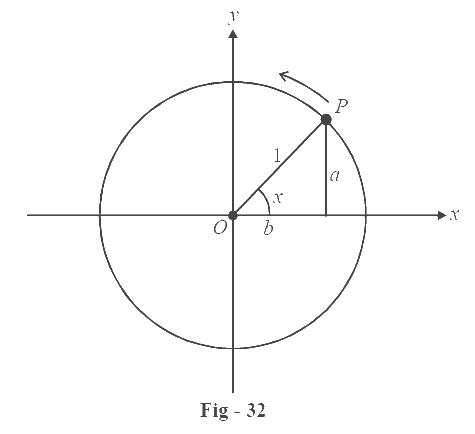We see that

\begin{align}\text{sin}x=\frac{a}{1}\end{align}=a

\begin{align}\text{cos}x=\frac{b}{1}\end{align}=b

\begin{align}\text{tan}x=\frac{a}{b}\end{align}

As the point P moves (x increases), note the variations in ‘a’ and ‘b’ and hence observe the details of the graphs we obtain.

(i) sin x (variation in ‘a’)

Domain = $$\mathbb{R}$$

Range= [–1, 1]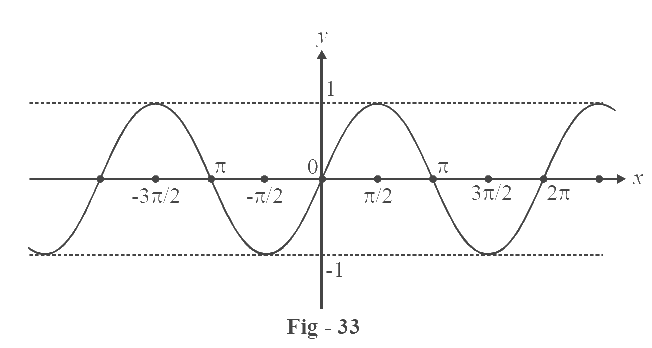The graph repeats after every complete revolution of P, i.e., after every increment(or decrement) of $$2\pi$$  in the angle ‘x’. This phenomenon is known as periodicity of the graph. We say that the graph is periodic with period ‘$$2\pi$$’.

(ii) cos x (variation in ‘b’)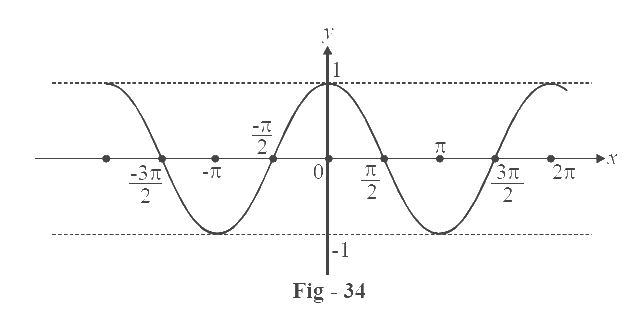Domain = $$\mathbb{R}$$

Range = [–1, 1]

This function is also periodic with period $$2\pi$$.

(iii) tan x (variation in ‘a/b’)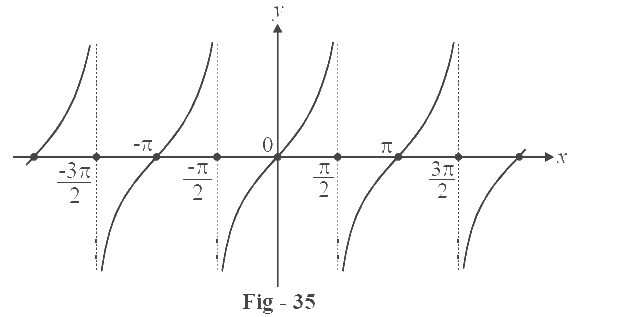Domain = $$\mathbb{R}$$ – \begin{align}\left\{ {n\pi + \frac{\pi }{2}} \right\}\end{align}

[Exclude the set of all points where b becomes 0, i.e. where the

angle x = n $$\pi$$ + $$\pi /2$$]

Range = $$\mathbb{R}$$

This function is periodic with period $$\pi$$. (half a revolution of P).

(iv) cosec x (variation in ‘1/a’)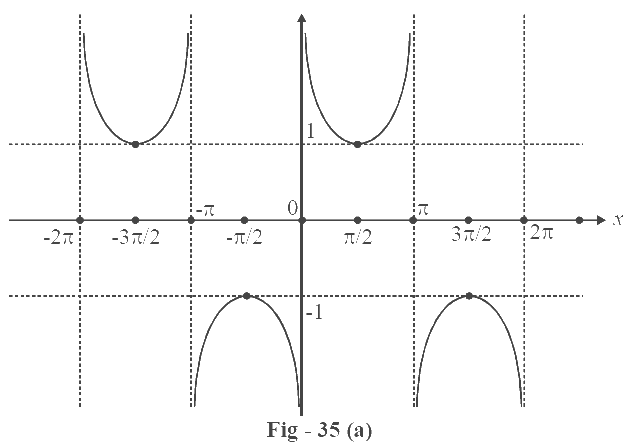$${\text{Domain}} = \mathbb{R} - \left\{ {n\pi } \right\},n \in \mathbb{Z}$$

$${\rm{Range}} = ( - \infty , - 1]\; \cup \;\;[1,\infty )$$

$$f\left( x \right) = {\rm{cosec}}\;x\;{\rm{is\;periodic\;with\;period\;2}}\pi$$

(v) sec x (variation in ‘1/b’)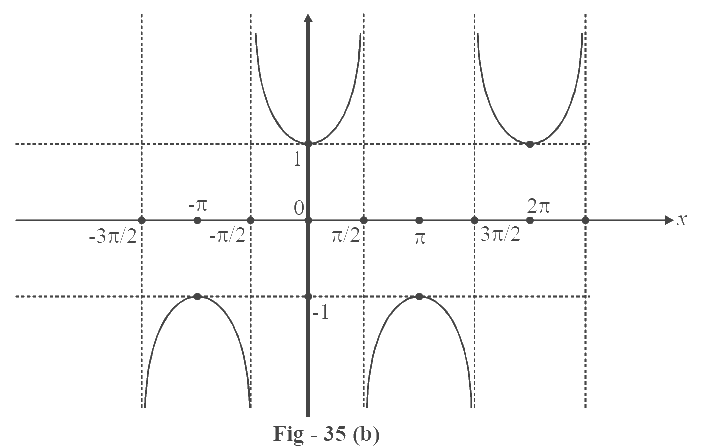\begin{align}{\text{Domain}} = \mathbb{R} - \left\{ {\left( {2n + 1} \right)\frac{\pi }{2}} \right\},\;n \in \mathbb{Z}\end{align}

[We exclude all points where cos x = 0, because \begin{align}\sec x = \frac{1}{{\cos x}}\end{align}]

$${\rm{Range}} = \left( { - \infty , - 1} \right]\; \cup \;\left[ {1,\infty } \right)\,$$

$$f\left( x \right) = \sec x\;{\rm{is\;again\;periodic\;with\;period\;2}}\pi {\rm{.}}$$

(vi) cot x (variation in ‘b/a’)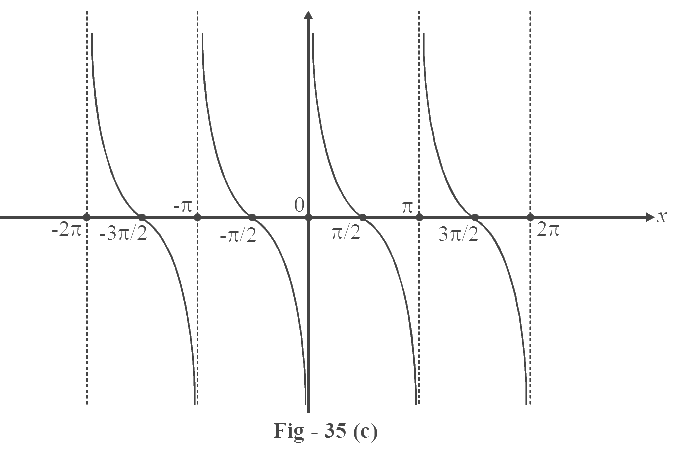$${\text{Domain}} = \mathbb{R} - \left\{ {n\pi } \right\},n \in \mathbb{Z}$$

$${\text{Range}} = \mathbb{R}$$

(x) = cot x is periodic with period $$\pi$$

Can you notice any relation between the graphs of reciprocal pairs?

$\sin x \leftrightarrow {\rm{cosec}}\;x, \qquad \cos x \leftrightarrow {\rm{sec}}\;x, \qquad \tan x \leftrightarrow \cot \;x$

If you can, there should be no problem in drawing the graph of say, cosec x from sin x, or, sec x from cos x etc.

In general, you will learn over time that plotting the graph of \begin{align}\frac{1}{{f\left( x \right)}}\end{align} from the graph of $$f\left( x \right)$$ is quite straightforward. You just have to kept the behavior of the reciprocal function in mind. And what is that behavior?

{\rm{If}}\;x > 0,\;{\rm{then}}\frac{1}{x} > 0: \left\{ {\;\begin{align}{{\rm{If}}\;x \to \infty ,}{\frac{1}{x} \to 0}\\{{\rm{If}}\;x \to 0,} {\frac{1}{x} \to \infty }\end{align}\;} \right\}

{\rm{If}}\;x < 0,\;{\rm{then}}\frac{1}{x} < 0: \left\{ {\begin{align}{\;{\rm{If}}\;x \to - \infty ,}{\frac{1}{x} \to 0}\\{{\rm{If}}\;x \to 0,} {\frac{1}{x} \to - \infty }\end{align}\;} \right\}

In case of any confusion, look at the graph of \begin{align}y = \frac{1}{x}\end{align}again.

12. Polynomials and polynomial ratios

Polynomial functions are function of the form $$f\left( x \right) = {a_0}{x^n} + {a_1}{x^{n - 1}} + ...... + {a_{n.}}$$ $$\left\{ \begin{gathered} n \in {\mathbb{Z}^ + } \hfill \\ {a_i} \in \mathbb{R} \hfill \\ \end{gathered} \right\}$$

They are continuous function (without any breaks in the graph; we will discuss the continuity of functions later) whose domain is $$\mathbb{R}$$ and range is $$\mathbb{R}$$ or a subset of $$\mathbb{R}$$.

For example               $$f\left( x \right) = {x^2} + 2x + 3\,$$ is a quadratic function

$$f\left( x \right) = a{x^3} + b{x^2} + cx + d$$is a cubic function.

Polynomial ratios are functions of the form \begin{align}h(x) = \frac{{f(x)}}{{g(x)}}\end{align}where f (x) and g(x) are themselves polynomials.

The domain will exclude all points where g(x) can become 0 (the roots of g(x)). The range will be a subset of  $$\mathbb{R}$$ (or $$\mathbb{R}$$). The continuity of such functions will be discussed later.